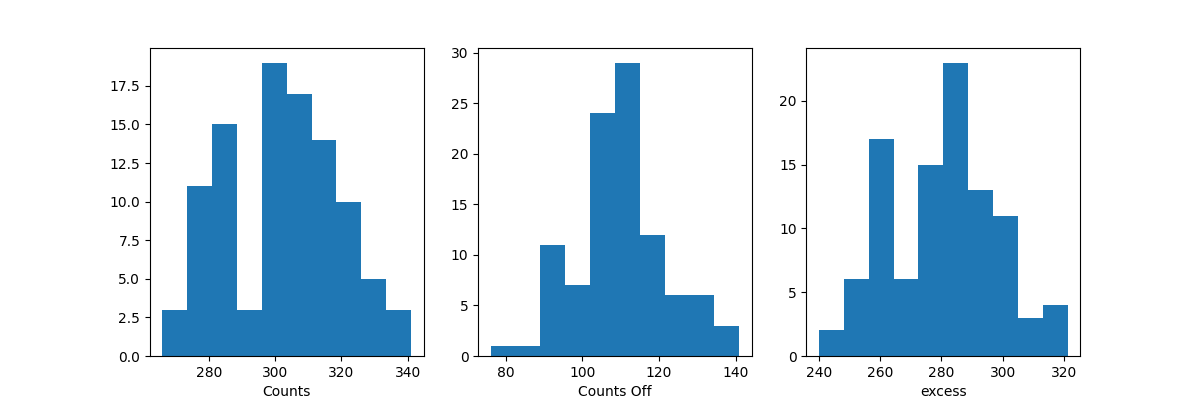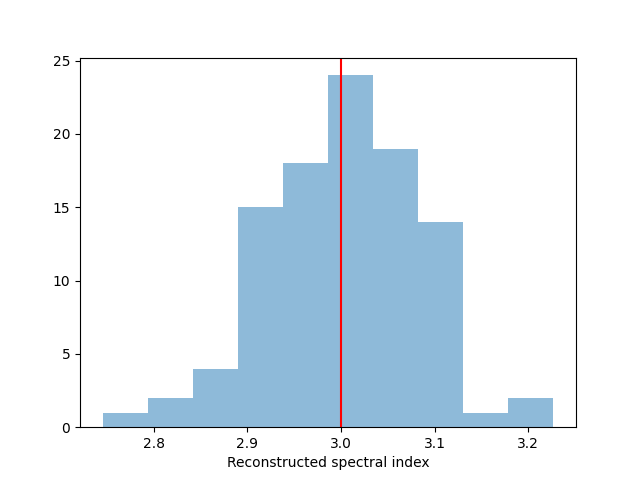# 1D spectrum simulation#

Simulate a number of spectral on-off observations of a source with a power-law spectral model using the CTA 1DC response and fit them with the assumed spectral model.

## Context#

To simulate a specific observation, it is not always necessary to simulate the full photon list. For many uses cases, simulating directly a reduced binned dataset is enough: the IRFs reduced in the correct geometry are combined with a source model to predict an actual number of counts per bin. The latter is then used to simulate a reduced dataset using Poisson probability distribution.

This can be done to check the feasibility of a measurement, to test whether fitted parameters really provide a good fit to the data etc.

Here we will see how to perform a 1D spectral simulation of a CTA observation, in particular, we will generate OFF observations following the template background stored in the CTA IRFs.

Objective: simulate a number of spectral ON-OFF observations of a source with a power-law spectral model with CTA using the CTA 1DC response, fit them with the assumed spectral model and check that the distribution of fitted parameters is consistent with the input values.

## Proposed approach#

We will use the following classes and functions:

```import numpy as np
import astropy.units as u
from astropy.coordinates import Angle, SkyCoord
from regions import CircleSkyRegion

# %matplotlib inline
import matplotlib.pyplot as plt
```

## Setup#

```from IPython.display import display
from gammapy.data import Observation, observatory_locations
from gammapy.datasets import Datasets, SpectrumDataset, SpectrumDatasetOnOff
from gammapy.makers import SpectrumDatasetMaker
from gammapy.maps import MapAxis, RegionGeom
from gammapy.modeling import Fit
from gammapy.modeling.models import PowerLawSpectralModel, SkyModel
```

## Check setup#

```from gammapy.utils.check import check_tutorials_setup

check_tutorials_setup()
```
```System:

python_executable      : /home/runner/work/gammapy-docs/gammapy-docs/gammapy/.tox/build_docs/bin/python
python_version         : 3.9.16
machine                : x86_64
system                 : Linux

Gammapy package:

version                : 1.1
path                   : /home/runner/work/gammapy-docs/gammapy-docs/gammapy/.tox/build_docs/lib/python3.9/site-packages/gammapy

Other packages:

numpy                  : 1.24.3
scipy                  : 1.10.1
astropy                : 5.2.2
regions                : 0.7
click                  : 8.1.3
yaml                   : 6.0
IPython                : 8.14.0
jupyterlab             : not installed
matplotlib             : 3.7.1
pandas                 : not installed
healpy                 : 1.16.2
iminuit                : 2.21.3
sherpa                 : 4.15.1
naima                  : 0.10.0
emcee                  : 3.1.4
corner                 : 2.2.2
ray                    : 2.5.0

Gammapy environment variables:

GAMMAPY_DATA           : /home/runner/work/gammapy-docs/gammapy-docs/gammapy-datasets/1.1
```

## Simulation of a single spectrum#

To do a simulation, we need to define the observational parameters like the livetime, the offset, the assumed integration radius, the energy range to perform the simulation for and the choice of spectral model. We then use an in-memory observation which is convolved with the IRFs to get the predicted number of counts. This is Poisson fluctuated using the `fake()` to get the simulated counts for each observation.

```# Define simulation parameters parameters
livetime = 1 * u.h

pointing = SkyCoord(0, 0, unit="deg", frame="galactic")
offset = 0.5 * u.deg

# Reconstructed and true energy axis
energy_axis = MapAxis.from_edges(
np.logspace(-0.5, 1.0, 10), unit="TeV", name="energy", interp="log"
)
energy_axis_true = MapAxis.from_edges(
np.logspace(-1.2, 2.0, 31), unit="TeV", name="energy_true", interp="log"
)

center = pointing.directional_offset_by(position_angle=0 * u.deg, separation=offset)

# Define spectral model - a simple Power Law in this case
model_simu = PowerLawSpectralModel(
index=3.0,
amplitude=2.5e-12 * u.Unit("cm-2 s-1 TeV-1"),
reference=1 * u.TeV,
)
print(model_simu)
# we set the sky model used in the dataset
model = SkyModel(spectral_model=model_simu, name="source")
```
```PowerLawSpectralModel

type      name     value         unit      ... max frozen is_norm link
-------- --------- ---------- -------------- ... --- ------ ------- ----
spectral     index 3.0000e+00                ... nan  False   False
spectral amplitude 2.5000e-12 cm-2 s-1 TeV-1 ... nan  False    True
spectral reference 1.0000e+00            TeV ... nan   True   False
```

Load the IRFs In this simulation, we use the CTA-1DC IRFs shipped with Gammapy.

```irfs = load_irf_dict_from_file(
"\$GAMMAPY_DATA/cta-1dc/caldb/data/cta/1dc/bcf/South_z20_50h/irf_file.fits"
)

location = observatory_locations["cta_south"]
obs = Observation.create(
pointing=pointing,
livetime=livetime,
irfs=irfs,
location=location,
)
print(obs)
```
```/home/runner/work/gammapy-docs/gammapy-docs/gammapy/.tox/build_docs/lib/python3.9/site-packages/astropy/units/core.py:2097: UnitsWarning: '1/s/MeV/sr' did not parse as fits unit: Numeric factor not supported by FITS If this is meant to be a custom unit, define it with 'u.def_unit'. To have it recognized inside a file reader or other code, enable it with 'u.add_enabled_units'. For details, see https://docs.astropy.org/en/latest/units/combining_and_defining.html
warnings.warn(msg, UnitsWarning)
/home/runner/work/gammapy-docs/gammapy-docs/gammapy/.tox/build_docs/lib/python3.9/site-packages/gammapy/data/observations.py:226: GammapyDeprecationWarning: Pointing will be required to be provided as FixedPointingInfo
warnings.warn(
Observation

obs id            : 0
tstart            : 51544.00
tstop             : 51544.04
duration          : 3600.00 s
pointing (icrs)   : 266.4 deg, -28.9 deg

```

Simulate a spectra

```# Make the SpectrumDataset
geom = RegionGeom.create(region=on_region, axes=[energy_axis])

dataset_empty = SpectrumDataset.create(
geom=geom, energy_axis_true=energy_axis_true, name="obs-0"
)
maker = SpectrumDatasetMaker(selection=["exposure", "edisp", "background"])

dataset = maker.run(dataset_empty, obs)

# Set the model on the dataset, and fake
dataset.models = model
dataset.fake(random_state=42)
print(dataset)
```
```SpectrumDataset
---------------

Name                            : obs-0

Total counts                    : 298
Total background counts         : 22.29
Total excess counts             : 275.71

Predicted counts                : 303.66
Predicted background counts     : 22.29
Predicted excess counts         : 281.37

Exposure min                    : 2.53e+08 m2 s
Exposure max                    : 1.77e+10 m2 s

Number of total bins            : 9
Number of fit bins              : 9

Fit statistic type              : cash
Fit statistic value (-2 log(L)) : -1811.58

Number of models                : 1
Number of parameters            : 3
Number of free parameters       : 2

Component 0: SkyModel

Name                      : source
Datasets names            : None
Spectral model type       : PowerLawSpectralModel
Spatial  model type       :
Temporal model type       :
Parameters:
index                         :      3.000   +/-    0.00
amplitude                     :   2.50e-12   +/- 0.0e+00 1 / (cm2 s TeV)
reference             (frozen):      1.000       TeV
```

You can see that background counts are now simulated

### On-Off analysis#

To do an on off spectral analysis, which is the usual science case, the standard would be to use `SpectrumDatasetOnOff`, which uses the acceptance to fake off-counts. Please also refer to simulations in the absence of a background model for simulations based on observations of real off counts.

```dataset_on_off = SpectrumDatasetOnOff.from_spectrum_dataset(
dataset=dataset, acceptance=1, acceptance_off=5
)
dataset_on_off.fake(npred_background=dataset.npred_background())
print(dataset_on_off)
```
```SpectrumDatasetOnOff
--------------------

Name                            : fCvdJIHi

Total counts                    : 304
Total background counts         : 21.20
Total excess counts             : 282.80

Predicted counts                : 302.46
Predicted background counts     : 21.09
Predicted excess counts         : 281.37

Exposure min                    : 2.53e+08 m2 s
Exposure max                    : 1.77e+10 m2 s

Number of total bins            : 9
Number of fit bins              : 9

Fit statistic type              : wstat
Fit statistic value (-2 log(L)) : 6.26

Number of models                : 1
Number of parameters            : 3
Number of free parameters       : 2

Component 0: SkyModel

Name                      : source
Datasets names            : None
Spectral model type       : PowerLawSpectralModel
Spatial  model type       :
Temporal model type       :
Parameters:
index                         :      3.000   +/-    0.00
amplitude                     :   2.50e-12   +/- 0.0e+00 1 / (cm2 s TeV)
reference             (frozen):      1.000       TeV

Total counts_off                : 106
Acceptance                      : 9
Acceptance off                  : 45
```

You can see that off counts are now simulated as well. We now simulate several spectra using the same set of observation conditions.

```n_obs = 100
datasets = Datasets()

for idx in range(n_obs):
dataset_on_off.fake(random_state=idx, npred_background=dataset.npred_background())
dataset_fake = dataset_on_off.copy(name=f"obs-{idx}")
dataset_fake.meta_table["OBS_ID"] = [idx]
datasets.append(dataset_fake)

table = datasets.info_table()
display(table)
```
``` name  counts       excess      ...   acceptance_off         alpha
...
------ ------ ----------------- ... ----------------- -------------------
obs-0    317 298.6000061035156 ...              45.0 0.20000000298023224
obs-1    275             253.0 ...              45.0                 0.2
obs-2    293             272.4 ...              45.0                 0.2
obs-3    280             257.6 ... 45.00000000000001 0.19999999999999998
obs-4    337             316.4 ...              45.0                 0.2
obs-5    283             258.6 ...              45.0                 0.2
obs-6    330             307.6 ... 44.99999999999999 0.20000000000000004
obs-7    283             257.2 ...              45.0                 0.2
obs-8    308             284.6 ...              45.0                 0.2
obs-9    299             278.6 ... 45.00000000000001 0.19999999999999998
...    ...               ... ...               ...                 ...
obs-90    286             267.0 ... 44.99999999999999 0.20000000000000004
obs-91    285             259.8 ...              45.0                 0.2
obs-92    313             289.4 ...              45.0                 0.2
obs-93    302             283.2 ...              45.0                 0.2
obs-94    322             300.2 ...              45.0                 0.2
obs-95    305             280.6 ... 44.99999999999999 0.20000000000000004
obs-96    301             277.4 ...              45.0                 0.2
obs-97    290             271.2 ...              45.0                 0.2
obs-98    301             280.6 ...              45.0                 0.2
obs-99    323             302.2 ...              45.0                 0.2
Length = 100 rows
```

Before moving on to the fit let’s have a look at the simulated observations.

```fix, axes = plt.subplots(1, 3, figsize=(12, 4))
axes.hist(table["counts"])
axes.set_xlabel("Counts")
axes.hist(table["counts_off"])
axes.set_xlabel("Counts Off")
axes.hist(table["excess"])
axes.set_xlabel("excess")
plt.show()
```Now, we fit each simulated spectrum individually

```results = []

fit = Fit()

for dataset in datasets:
dataset.models = model.copy()
result = fit.optimize(dataset)
results.append(
{
"index": result.parameters["index"].value,
"amplitude": result.parameters["amplitude"].value,
}
)
```

We take a look at the distribution of the fitted indices. This matches very well with the spectrum that we initially injected.

```fig, ax = plt.subplots()
index = np.array([_["index"] for _ in results])
ax.hist(index, bins=10, alpha=0.5)
ax.axvline(x=model_simu.parameters["index"].value, color="red")
ax.set_xlabel("Reconstructed spectral index")
print(f"index: {index.mean()} += {index.std()}")
plt.show()
``````index: 3.0036925550408533 += 0.08081469527207054
```

## Exercises#

• Change the observation time to something longer or shorter. Does the observation and spectrum results change as you expected?

• Change the spectral model, e.g. add a cutoff at 5 TeV, or put a steep-spectrum source with spectral index of 4.0

• Simulate spectra with the spectral model we just defined. How much observation duration do you need to get back the injected parameters?

Gallery generated by Sphinx-Gallery